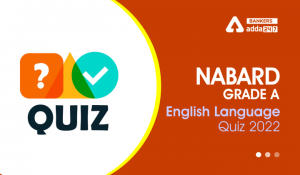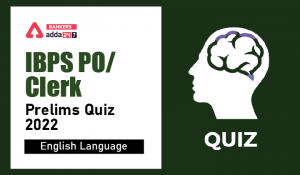Latest Banking jobs   »   Basic English Language Quiz for All...

# Basic English Language Quiz for All Banking Exams- 6th May

Directions (1-15): Find out the error in each of the following sentences, if there is no error, answer is (e). Avoid punctuation mistakes (if any).

Q1. (a) If she will work/ (b) hard she/(c) will surely / (d) clear the exam. / (e) No error
(a) a
(b) b
(c) c
(d) d
(e) e

Q2. (a) Before the teacher /(b) will come you / (c) should better / (d) complete your test. / (e) No error
(a) a
(b) b
(c) c
(d) d
(e) e

Q3. (a) If you /(b) asked him, /(c) he would / (d) help you./ (e) No error
(a) a
(b) b
(c) c
(d) d
(e) e

Q4. (a) Until the bus/(b) will not get the signal, /(c) it will not / (d) leave the stand. / (e) No error
(a) a
(b) b
(c) c
(d) d
(e) e

Q5. (a) If she had money/ (b) she will have/ (c) lent it/ (d) to me. / (e) No error
(a) a
(b) b
(c) c
(d) d
(e) e

Q6. (a) If I was you/ (b) I would not/(c) tolerate her / (d) for a moment. / (e) No error
(a) a
(b) b
(c) c
(d) d
(e) e

Q7. (a) Suppose he/(b) does not attend the meeting/ (c) what could/ (d) we do ? / (e) No error
(a) a
(b) b
(c) c
(d) d
(e) e

Q8. (a) Supposing if he /(b) does not reach / (c) the examination centre in time, / (d) what will his teacher do? / (e) No error
(a) a
(b) b
(c) c
(d) d
(e) e

Q9. (a) If you saw / (b) a ghost what / (c) will your/ (d) reaction be?/ (e) No error
(a) a
(b) b
(c) c
(d) d
(e) e

Q10. (a) If I had/(b) two cars, / (c) I would have given / (d) one to you./ (e) No error
(a) a
(b) b
(c) c
(d) d
(e) e

Q11. (a) If we will/(b) heat camphor / (c) it turns / (d) to vapour. / (e) No error
(a) a
(b) b
(c) c
(d) d
(e) e

Q12. (a) Suppose if you are / (b) late, you will not be / (c) allowed to enter the examination hall. / (e) No error.
(a) a
(b) b
(c) c
(d) d
(e) e

Q13. (a) Unless / (b) he will not understand/(c) the importance of this project/ (c) he will not /(d) work sincerely. / (e) No error
(a) a
(b) b
(c) c
(d) d
(e) e

Q14. (a) If he tried again,/ (b) he can win/(c) the race/ (d) with decent margin. / (e) No error
(a) a
(b) b
(c) c
(d) d
(e) e

Q15. (a) I will wait/ (b) for her / (c) unless / (d) she comes. / (e) No error
(a) a
(b) b
(c) c
(d) d
(e) e

Practice More Questions of English for Competitive Exams:

###### Basic English Study Plan for All Banking Exams

Solutions

S1. Ans. (a)
Sol. Change ‘If she will work hard’ into ‘If she works hard ‘. ‘Will’ is not used in the ‘If clause’.

S2. Ans. (b)
Sol. Change ‘before the teacher will come’ into ‘before the teacher comes ‘.

S3. Ans. (e)
Sol. No error

S4. Ans. (b)
Sol. Remove ‘will not’. ‘Not’ and ‘will’ do not come after ‘unless’. Change ‘get’ into ‘gets’

S5. Ans. (b)
Sol. Change ‘will have lent’ into ‘would lend’.

S6. Ans. (a)
Sol. Change ‘was’ into ‘were’. ‘Was’ is not used in imaginary sentence.

S7. Ans. (c)
Sol. Change ‘could’ into ‘can’. The sentence is not of past ability.

S8. Ans. (a)
Sol. Remove ‘if’ .’supposing’ and ‘if’ mean the same. When used together, they make the sentence superfluous.

S9. Ans. (c)
Sol. Change ‘will’ into ‘would’.

S10. Ans. (c)
Sol. Change ‘I would have given’ into ‘I would give’.

S11. Ans. (a)
Sol. Remove ‘will’.

S12. Ans. (a)
Sol. Remove ‘if.’ ‘Suppose’ and ‘if’ mean the same. When used together, they make the sentence superfluous.

S13. Ans. (b)
Sol. Change ‘he will not understand’ into ‘he understands’. ‘Not’ and ‘will’ do not come after ‘unless’.

S14. Ans. (b)
Sol. Change ‘can’ into ‘could’. The sentence is in past.

S15. Ans. (c)
Sol. Change ‘unless’ into ‘until’. Until is time oriented whereas unless is action oriented.

#### Congratulations!Incorrect details? Fill the form again here

•English Quizzes For NABARD Grade A 2022-...
•English Quizzes For IBPS Clerk/PO Prelim...
•English Quizzes For NABARD Grade A 2022-...
•English Quizzes For IBPS Clerk/PO Prelim...
•English Quizzes For NABARD Grade A 2022-...
•English Quizzes For IBPS Clerk/PO Prelim...